Padua points (named after the University of Padua, where they were discovered in 2005 ) are the first known example of a unisolvent point set over bivariate polynomials which have a provably minimal growth in Lebesgue constant of $O(\log^2 n)$. They are sometimes refered to as the "Chebyshev points in 2D" .

It seemed only natural to include these points in Chebfun, and they are now available through the paduapts method. For example, here is the Padua grid corresponding to $n = 8$:

n = 8;
plot(x(:,1), x(:,2), 'ok', 'MarkerFaceColor', 'k')
axis equal, axis([-1 1 -1 1]), hold on
FS = 'FontSize';
title('Padua points', FS, 14), set(gca, FS, 12)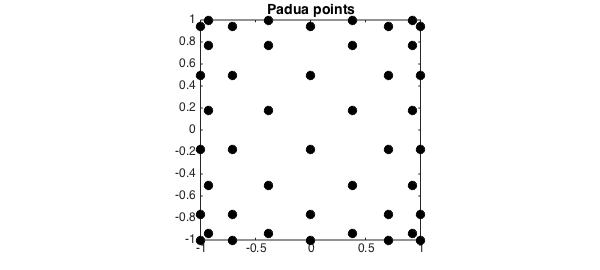There are a number of characterizations of Padua points. One is as the intersection of a certain Lissajous curve  with itself and the boundary of $[-1,1]\times[-1,1]$:

t = chebfun('t', [0 pi]);
L = -cos((n + 1)*t) - 1i*cos(n*t);        % Lissajous curve
plot(L, 'b')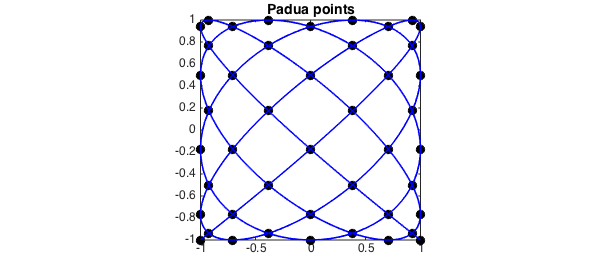Another is as every other point from an $(n+1)\times(n+2)$ tensor product Chebyshev grid:

x1 = chebpts(n + 1);
x2 = chebpts(n + 2);
[X, Y] = meshgrid(x1, x2);
plot(X, Y, 'or'), hold off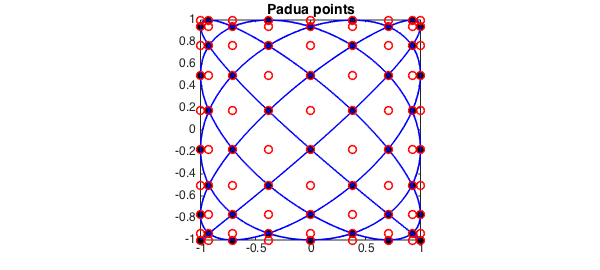If a matrix rather than a bivariate function is supplied as an input, Chebfun2 normally assumes that its values correspond to data on a Chebyshev tensor product grid. However, if the 'padua' flag is supplied, the constructor assumes the values are data on a Padua grid and returns a bivariate polynomial that interpolates the prescribed data. For example:

f = @(x,y) cos(exp(2*x+y)).*sin(y);
fx = f(x(:,1), x(:,2));                   % Samples from Padua grid
F = chebfun2(fx, [-1 1 -1 1], 'padua');   % Construct Chebfun2 from samples
plot(F), hold on
plot3(x(:,1), x(:,2), F(x(:,1), x(:,2)), 'ok', 'MarkerFaceColor', 'k'), hold off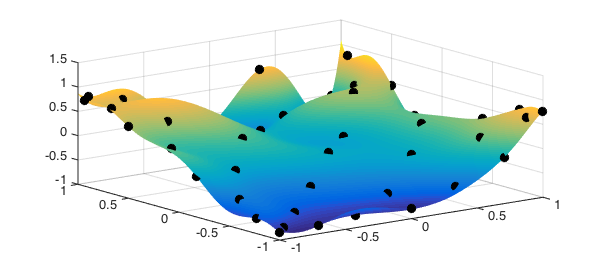The corresponding Padua interpolant is a bivariate Chebyshev polynomial of total degree $n$ (i.e. the degrees in $x$ and $y$ sum to at most $n$). We can verify this by looking at the coefficients of the interpolant:

C = chebcoeffs2(F);
C(abs(C) < 1e-10) = 0;
spy(C), shg
title('Spy plot of bivariate Chebyshev coefficients',FS,14)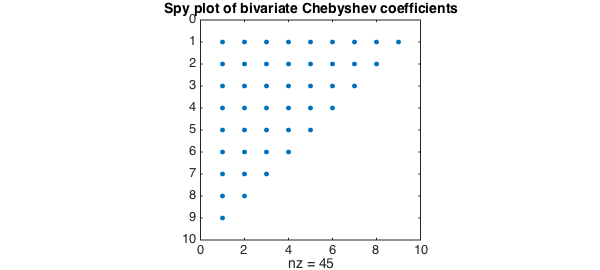We hope this addition will make it easy to explore Padua interpolants in Chebfun2!

1. M. Caliari, S. De Marchi, and M. Vianello, "Bivariate polynomial interpolation on the square at new nodal sets", Applied Mathematics and Computation, 165 (2005), 261-274.

2. Chebfun Example: geom/Lissajous